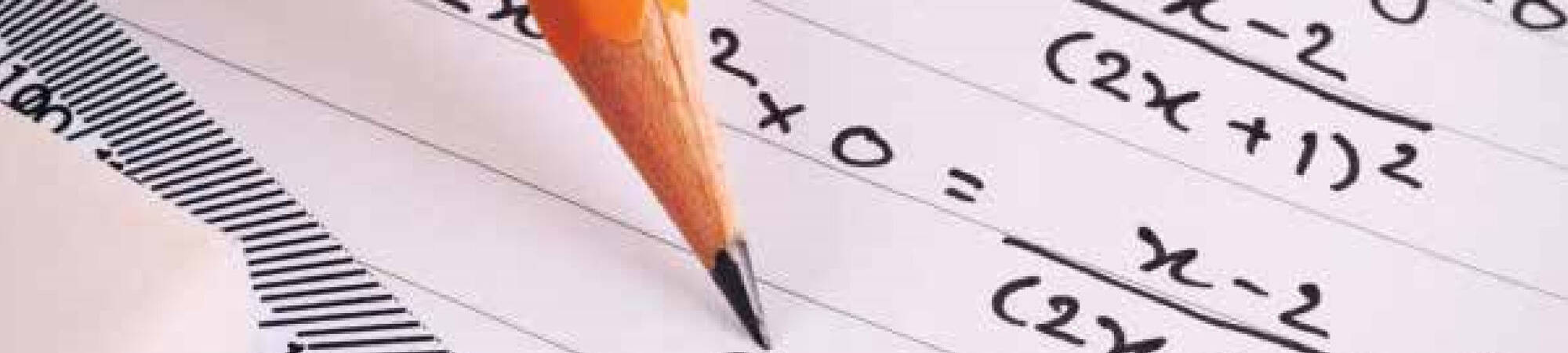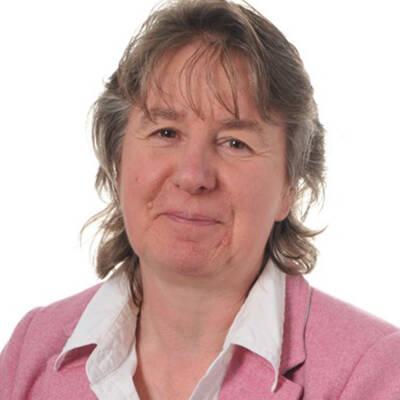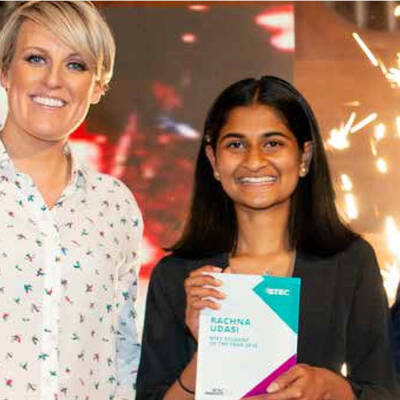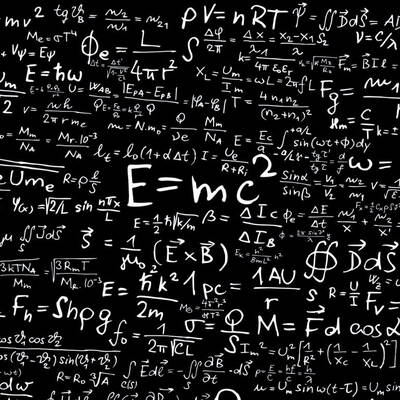# Mathematics

## Exam Body

Pearson (A Level)

Jillian Dolder

Mathematics offers a means of looking at patterns that make up our world and the intricate and beautiful ways in which they are constructed.#### A Level

Entry for the A Level course requires the ability and the desire to take the subject well beyond GCSE Level, even for AS Level Mathematics. Typically, students with a grade 7 or higher in Mathematics GCSE/iGCSE are best suited to this course.

Mathematics at A Level is more stimulating than at GCSE. Students will be challenged and given the tools they will need to succeed in their further adventures in Mathematics. The main emphasis is on learning techniques and skills, understanding concepts, and applying these to solving problems.

A Level Mathematics is highly regarded by Universities of all disciplines and, as such, complements a wide range of subjects when studied as a block. Students will acquire skills and knowledge that can be applied in many fields; developing their powers of reason and logic, as well as communication. Mathematics is key to pursuing a career in Physics, Engineering, or as a foundation when aiming to study, Banking, Business Studies, or Economics at a Higher Level.

Universities and employers’ value the academic training that Mathematics provides and areas such as Law or Computing consider the logical training provided by Mathematics as extremely helpful.

St David's is where you are encouraged, supported, nurtured yet stretched to achieve potential that you didn't know you have!

## A-Level Course Content

The Mathematics A Level course is a linear course, consisting of three 2-hour papers for the A Level award.

Pure Mathematics is the study of abstract topics such as geometry, algebra, trigonometry, functions, and calculus. It includes Indices and surds; Polynomials; Coordinate geometry and graphs; Trigonometry; Sequences and series; Algebra and functions; Numerical methods; Differentiation and integration; Differential equations; Vectors.

Applied Mathematics relates the knowledge and skills learned in Pure Mathematics to real-life situations and is broken down into two areas, Statistics and Mechanics.

• Mechanics is the study of forces and moving objects; Mechanics Forces as a vector; Equilibrium of a particle; Kinematics of motion in a straight line; Newton’s Laws of Motion; Linear momentum.
• Statistics is the study of probability and the analysis of data. Statistics Representation of data; Probability; Discrete random variables; Normal and Binomial Distribution along with hypothesis testing.